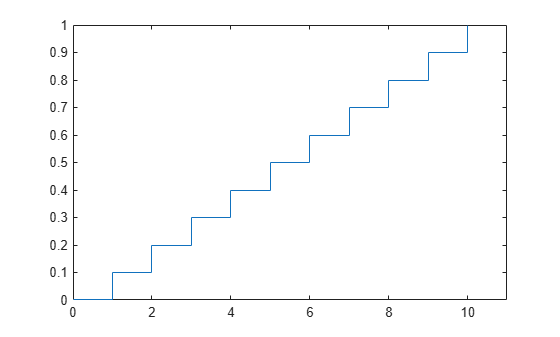Uniform Distribution (Discrete)

Definition

The discrete uniform pdf is

$y=f\left(x|N\right)=\frac{1}{N}{I}_{\left(1,...,N\right)}\left(x\right)$

Background

The discrete uniform distribution is a simple distribution that puts equal weight on the integers from one to N.

Examples

Plot a Discrete Uniform Distribution cdf

As for all discrete distributions, the cdf is a step function. The plot shows the discrete uniform cdf for N = 10.

x = 0:10;
y = unidcdf(x,10);
figure;
stairs(x,y)
h = gca;
h.XLim = [0 11];Generate Discrete Uniform Random Numbers

Pick a random sample of 10 from a list of 553 items.

rng default;  % for reproducibility
numbers = unidrnd(553,1,10)
numbers = 1×10

451   501    71   506   350    54   155   303   530   534